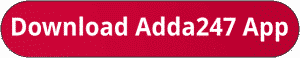Latest Banking jobs   »   Bank Foundation quant

# Quantitative Aptitude Quiz For Bank Foundation 2023-14th November

Directions (1-5): In each of the following questions two equations are given. Solve these equations and give answer:
(a) if x≥y, i.e. x is greater than or equal to y
(b) if x>y, i.e. x is greater than y
(c) if x≤y, i.e. x is less than or equal to y
(d) if x<y, i.e. x is less than y
(e) x=y or no relation can be established between x and ySolutions.                                                           .                                           .      .

.                      .                                           .                                      .                                    .                                      .                            .                                   .                                   .                      .                     .

## FAQs

### What is the selection process of the Bank Clerk?

The selection process of the Bank Clerk is Prelims & Mains.

#### Congratulations!Union Budget 2023-24: Free PDF